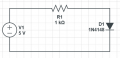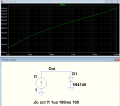# Does a diode influence the current of a circuit?

#### jimmy_newbie

Joined Nov 24, 2022
8
Hello!
I'm trying to learn the behaviour of a diode. In particular, I would like to know if it is able to set the current on its branch.
As far as I understood, once the diode is on, its voltage drop $V_D$ should be constant, regardless of the rest of the circuit: so, the diode should "decide" to "absorb" a specific amount of current, such that the voltage drop on itself keeps being a specific value. This value should also be equal to the threshold voltage of the diode: so, $V_D = V_{\mathrm{th}}$
I tried to simulate the simple attached circuit, with a DC voltage generator, a resistor and the 1N4148 diode with SPICE:When R1 = 1k, I obtained a voltage drop across the diode

$V_D = 677.2 \ \mathrm{mV}$

with a 4.323 mA current. From the datasheet of the diode, I can not clearly understand what should be the threshold voltage of this diode. However, if I try to raise the resistor R1 value, I obtain each time different voltage drops across the diode: they become smaller.

With R1 = 10k,

$V_D = 565.8 \ \mathrm{mV}$

With R1 = 100k,

$V_D = 456.7 \ \mathrm{mV}$

and of course the current is smaller each time.

Why does this happen? Shouldn't the diode try to absorb a current such that its V_D keeps being constant (with minor variations due to the non-ideal components)?
Who sets the current in this circuit?

Instead, if I keep R1 constant and make a DC sweep analysis for V1 from (for example) 0 to 20 V, once the diode is on, the current and the voltage drop across the diode only slightly grow, as V1 raises, showing the behaviour I was expecting. Why varying V1 it's different than varying R1?

Jimmy

#### BobTPH

Joined Jun 5, 2013
6,095
As far as I understood, once the diode is on, its voltage drop VDshould be constant, regardless of the rest of the circuit:
Where did you get that idea?

The voltage across a diode increases with any increase in current. It just doesn’t change linearly with the current, it changes logarithmically. Or, alternatively, the current rises exponentially with increasing voltage.

#### Alec_t

Joined Sep 17, 2013
13,237
As a rule of thumb, when designing a circuit, the voltage drop across a common silicon diode is usually taken to be about 0.6V.
A Schottky diode voltage drop is about 0,3V. These are approximations only.

•DickCappels

#### DickCappels

Joined Aug 21, 2008
9,524
If you don't mind looking at hairy equations you might find improved understanding by looking at the relationships on this Wiki page:
https://en.wikipedia.org/wiki/Diode_modelling

This information will also be helpful in understanding transistors.

•jimmy_newbie

#### crutschow

Joined Mar 14, 2008
31,151
As far as I understood, once the diode is on, its voltage drop should be constant, regardless of the rest of the circuit
Not so, grasshopper.
Reverse-biased Zener diodes have a fairly constant voltage drop versus current, but not forward biased junction diodes.

Below is the simulation of the diode voltage versus forward current.
It's plotted with a horizontal log scale for the current, which gives close to a straight line for the voltage rise, showing the diode's log relation between voltage and current.

A 0.6-0.7V diode drop is often assumed for design purposes, which is for a typical current range of diodes used in small signal circuits (1-10mA).Last edited:
•jimmy_newbie

#### MrChips

Joined Oct 2, 2009
27,727
•jimmy_newbie

#### WBahn

Joined Mar 31, 2012
27,942
As others have said, the voltage across a diode increases as the current through it increases (just to foreshadow you, you'll discover that there are special types of diodes, such as tunnel diodes. for which this is not true over some regions of operation and the current voltage actually goes down as the current goes up, thus exhibiting negative resistance in that region -- a trait that has been exploited in many designs).

There are several mathematical models for diodes and, in general, we use the simplest one that gives us an acceptably accurate result for what we are interested in.

The simplest model is the "ideal" diode that has zero voltage across it when forward biases no matter how much current is flowing and has zero current flowing through it no matter how much reverse voltage is across it. This model is very simple to use and in many situations gets us a result that is "good enough".

The next model, which is probably the most widely used model, is the "constant-voltage" diode model. That is the model you are using. This model is essentially the same as the ideal diode model except that the diode has a threshold voltage that must be reached before conduction occurs. It is so widely used that people often mistakenly refer to THIS model as the ideal diode model, so sometimes you need to be careful about interpreting what is meant. The threshold voltage is taken to be a constant for a given type of diode, with silicon diodes usually being taken as something between 0.6 V and 0.7 V, while other types of diodes, such as germanium, might be in the 0.3 V range.

We can use this model whenever we can say that the actual voltage drop across the diode has little impact on circuit behavior. So, for a silicon diode, if the voltage turns out to be 0.5 V or 0.8 V and the results are still "good enough", then they are good enough. In most situations, if this is not the case, it is an indication that we need to speed more time improving the circuit design to make it so that it is the case.

The next step up in diode model is usually the Schockley model, which is

$$i_D \; = \; I_S \left( e^{\frac{v_d}{nV_T}} \; - \; 1\right)$$

V_T is the "thermal voltage" and is equal to kT/q (look it up for more detail) which is about 26 mV at room temperature.

The n is the "ideality factor" (there are some other names that are used). For transistors (which also use this model for the collector current as a function of base-emitter voltage), n is almost always close enough to 1 that we ignore it, but in diodes it is is more variable and can be closer to 2 (which is commonly just assumed to be the value). Note, again, those words "close enough" -- engineering is all about solutions that are "close enough". It is often the case that just always assuming n=1 is close enough.

That "-1" in the equation is almost always ignored. It's there to ensure that the model predicts that there is zero current flowing when there is zero voltage across the device. The value of Is (the so-called "reverse saturation current") is so small (typically picoamperes) that ignoring the -1 almost never has any significance.

Note that the Schockley model is NOT the end-all, be-all for diode modeling. It is an approximation that works very well for many types of PN junctions, but not all of them. For instance, very shallow junctions often need better models.

It also doesn't account for ohmic resistance, which can be a very important part in the behavior of real diodes due to their construction. So other models incorporate that.

•jimmy_newbie

#### jimmy_newbie

Joined Nov 24, 2022
8
Below is the simulation of the diode voltage versus forward current.
[...]
A 0.6-0.7V diode drop is often assumed for design purposes, which is for the typical current range of diodes used in small signal circuits.
Thanks for your observations and for the simulation. It has been very useful and it's more clear, now!

#### jimmy_newbie

Joined Nov 24, 2022
8

[...] The next model, which is probably the most widely used model, is the "constant-voltage" diode model. That is the model you are using. [...] The threshold voltage is taken to be a constant for a given type of diode, with silicon diodes usually being taken as something between 0.6 V and 0.7 V, while other types of diodes, such as germanium, might be in the 0.3 V range.
Yes, and also answering BobTPH, I unintentionally took this model as the only one.

[...] The next step up in diode model is usually the Schockley model
[...]
Note that the Schockley model is NOT the end-all, be-all for diode modeling. It is an approximation that works very well for many types of PN junctions, but not all of them. For instance, very shallow junctions often need better models.
It's far better now. The Schockley model is the reason for the plots in crutschow answer.
Thanks also for showing the limits of the models, to be more aware about when they can be used.

#### WBahn

Joined Mar 31, 2012
27,942
I actually overlooked another model that can be used that would go between the constant-voltage model and the Schockley model, and that's the piecewise-linear model. Here we put a resistor in series with the a voltage source to capture an approximation of the increase in voltage with current. The tricky part is balancing the resistance against the voltage to get an acceptable fit to the exponential curve. I don't think I've ever used this model outside of the classroom (which is why I neglected it). Usually, if this is important, you either use the Schockley model or you break your analysis into a superposition of a DC bias point solution and an AC small-signal solution about that bias point.

•jimmy_newbie